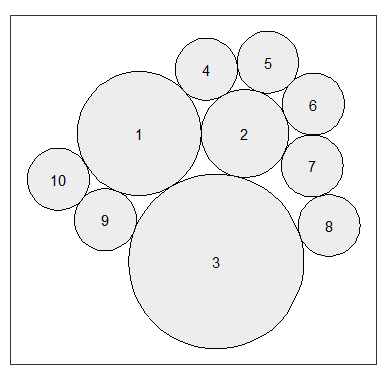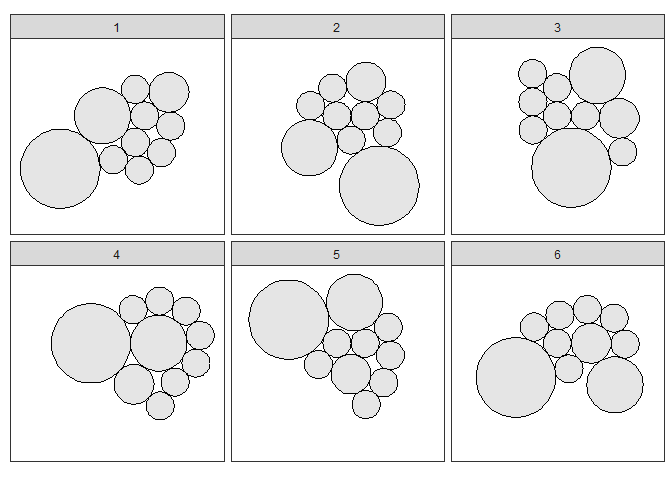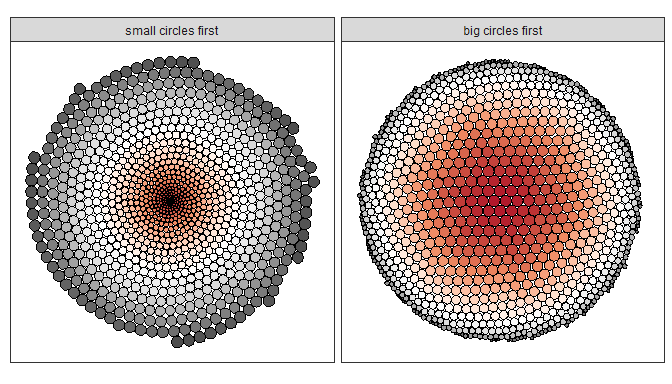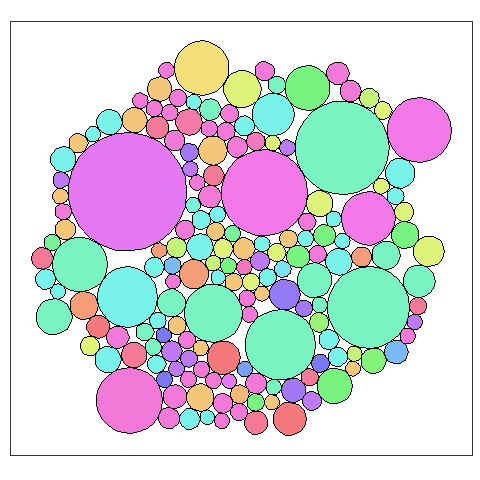# Progressive circle packing

#### 2022-11-22

The function circleProgressiveLayout arranges a set of circles deterministically. The first two circles are placed to the left and right of the origin respectively. Subsequent circles are placed so that each:

• is externally tangent to two previously placed circles;
• does not overlap another circle;
• and is as close as possible to the origin.

The algorithm was described in the paper: Visualization of large hierarchical data by circle packing by Weixin Wang et al. (2006). The implementation in this package is based on a version written in C by Peter Menzel.

The algorithm is very efficient and this, combined with the implementation in Rcpp, means arrangements for large numbers of circles can be found quickly.

## First example

We begin by arranging 10 circles of various sizes. First we pass a vector of circle areas to the circleProgressiveLayout function. It returns a data frame of centre coordinates and radii.

areas <- c(20, 10, 40, rep(5, 7))

# Generate the layout
packing <- circleProgressiveLayout(areas)

head( round(packing, 2) )
##       x     y radius
## 1 -2.52  0.00   2.52
## 2  1.78  0.00   1.78
## 3  0.61 -5.22   3.57
## 4  0.22  2.61   1.26
## 5  2.72  2.90   1.26
## 6  4.59  1.19   1.26

Next we derive a data frame of circle vertices for plotting using the circleLayoutVertices function, and then use ggplot to display the layout, labelling the circles to show their order of placement:

library(ggplot2)

t <- theme_bw() +
theme(panel.grid = element_blank(),
axis.text=element_blank(),
axis.ticks=element_blank(),
axis.title=element_blank())

theme_set(t)

dat.gg <- circleLayoutVertices(packing, npoints=50)

ggplot(data = dat.gg) +
geom_polygon(aes(x, y, group = id), colour = "black",
fill = "grey90", alpha = 0.7, show.legend = FALSE) +

geom_text(data = packing, aes(x, y), label = 1:nrow(packing)) +

coord_equal()By default, circleProgressiveLayout takes the input sizes to be circle areas. If instead you have input radii, add sizetype = "radius" to the arguments when calling the function.

## Layouts are order dependent

Re-ordering the input sizes will generally produce a different layout unless the sizes are uniform. Here we repeatedly shuffle the area values used above and generate a new layout each time.

ncircles <- length(areas)
nreps <- 6

packings <- lapply(
1:nreps,
function(i) {
x <- sample(areas, ncircles)
circleProgressiveLayout(x)
})

packings <- do.call(rbind, packings)

npts <- 50
dat.gg <- circleLayoutVertices(packings, npoints = npts)

dat.gg$rep <- rep(1:nreps, each = ncircles * (npts+1)) ggplot(data = dat.gg, aes(x, y)) + geom_polygon(aes(group = id), colour = "black", fill = "grey90") + coord_equal() + facet_wrap(~ rep, nrow = 2)We can use this ordering effect to create some circle art… areas <- 1:1000 # area: small to big packing1 <- circleProgressiveLayout(areas) dat1 <- circleLayoutVertices(packing1) # area: big to small packing2 <- circleProgressiveLayout( rev(areas) ) dat2 <- circleLayoutVertices(packing2) dat <- rbind( cbind(dat1, set = 1), cbind(dat2, set = 2) ) ggplot(data = dat, aes(x, y)) + geom_polygon(aes(group = id, fill = -id), colour = "black", show.legend = FALSE) + scale_fill_distiller(palette = "RdGy") + coord_equal() + facet_wrap(~set, labeller = as_labeller( c('1' = "small circles first", '2' = "big circles first")) )## More detailed layout display The package includes an example data set of the abundance of different types of bacteria measured in a study of biofilms. Columns are value (abundance), display colour and label (bacterial taxon). data("bacteria") head(bacteria) ## value colour label ## 1 4232 #DDF379 Dehalogenimonas sp. WBC-2 ## 2 5097 #F3C679 Desulfatibacillum alkenivorans AK-01 ## 3 2825 #79F398 Opitutus terrae PB90-1 ## 4 3471 #F3C679 Geobacter ## 5 7515 #79F3EA Methanosaeta harundinacea 6Ac ## 6 2476 #C4F379 Rubinisphaera brasiliensis DSM 5305 The following example shows how to display the abundance values as circles filled with the specified colours. It relies on the fact that the id column in the output of circleLayoutVertices maps to the row number of the input data. Note: the y-axis is reversed so that the layour is rendered similarly to the example here. packing <- circleProgressiveLayout(bacteria) dat.gg <- circleLayoutVertices(packing) ggplot(data = dat.gg) + geom_polygon(aes(x, y, group = id, fill = factor(id)), colour = "black", show.legend = FALSE) + scale_fill_manual(values = bacteria$colour) +

scale_y_reverse() +

coord_equal()As a further flourish, we can make the plot interactive so that the name of the bacterial taxon is displayed when the mouse cursor hovers a circle.

Note: the ggiraph package is required for this.

if (requireNamespace("ggiraph")) {

gg <- ggplot(data = dat.gg) +
ggiraph::geom_polygon_interactive(
aes(x, y, group = id, fill = factor(id),
tooltip = bacteria$label[id], data_id = id), colour = "black", show.legend = FALSE) + scale_fill_manual(values = bacteria$colour) +

scale_y_reverse() +

labs(title = "Hover over circle to display taxon name") +

coord_equal()

ggiraph::ggiraph(ggobj = gg, width_svg = 5, height_svg = 5)

}
## Loading required namespace: ggiraph